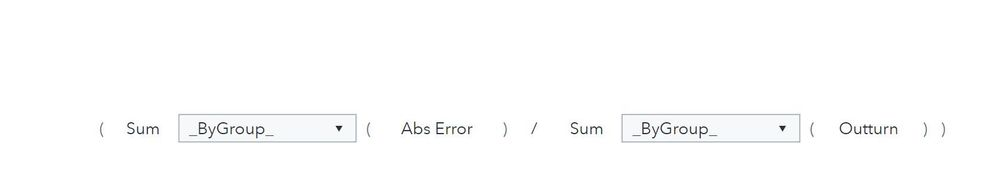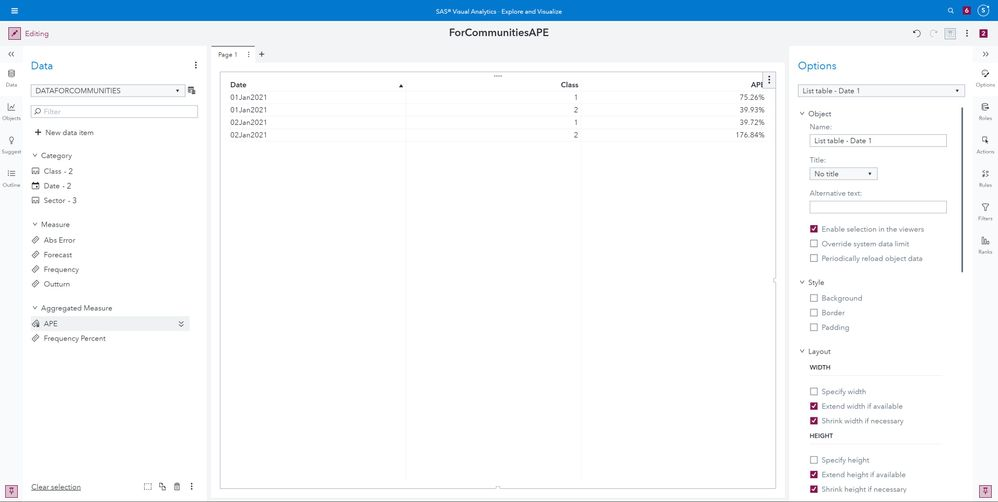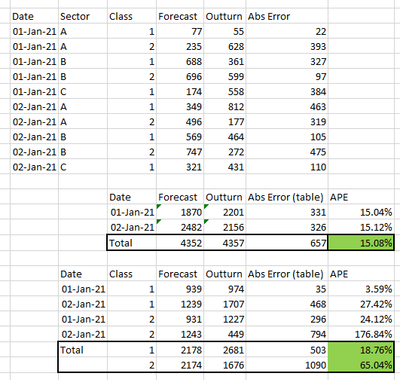## visual analytics percentage error calculation

Hi,

I am trying to do a calculation of forecast performance in visual analytics.

The answer I need is a nuanced version of the mean absolute percentage error but hopefully this example will explain.In this spreadsheet i have some random data by date and some other groupings with forecasts and what the outturn value was, and 3 different ways of calculating the absolute percentage error.
option 1 I can do in VA by doing a sum by group abs(forecast-outturn) but you can see that because I have different sectors etc the percentage is way too high.
option 2 I can do by doing abs(sum by group forecast - sum by group outturn) but this is way too low because the errors are netting out between the different days.

option 3 is what I need but I don't know how to get VA to do the sum by date first and then work out the total as a second step.

I know that one option would be to use aggregated tables to get the totals by date and then calculate from there but for the final report we are interested how it differs if I filter on just a specific sector or class so I need it to be more flexible.

If anyone has any insight on this it would be much appreciated.

Tim

2 REPLIES 2

## Re: visual analytics percentage error calculation

Would this work? I created a calculated Item named APE## Re: visual analytics percentage error calculation

No, that one is summing all the individual absolute errors so it's overstating the error.

here is an updated view of the spreadsheet with the calculations for how the grouping should break down. (also corrected the sums pointing to the wrong range on one of them)the calculation I am after is...

Abs Error = abs ( Sum (By [Class/Sector/Ect.] and Date) Forecast  -  Sum (By [Class/Sector/Ect.] and Date) Outturn )

MAPE = Sum (By [Class/Sector/Ect.]) Abs Error / Sum (By [Class/Sector/Ect.]) Outturn

but VA won't let you put the aggregation Abs Error into another aggregation.

Discussion stats
• 2 replies
• 488 views
• 0 likes
• 2 in conversation